Vibroengineering PROCEDIA

Published: 28 November 2019

# Simulation on metro railway induced vibration. Part II: effect of corrugated rail

Hougui Zhang1
Zhou Ren2
Qiong Wu3
Jie Yang4
1, 3, 4Beijing Municipal Institute of Labour Protection, Beijing 100054, China
2Beijing Jiaotong University, Beijing 100044, China
Corresponding Author:
Hougui Zhang
Views 132

#### Abstract

The deterioration of running surface, including out-of-round wheels and corrugated rails. The effect of out-of-round wheels on simulation of rail dynamic behavior was presented in a companion paper . In this paper, the effect of corrugated rails was discussed. Considering a perfect round wheels moving on different irregularities rail surface, including perfect smooth rail, irregularities generated from a US PSD, measurement data from corrugated rails. Calculation result indicated that (1) the traditional used irregularity generated from US-PSD would not satisfy the requirement simulation on rolling noise and vibration generation in high frequency range; (2) corrugated rail plays the dominant role on the vibration which obviously related to its typical wavelength.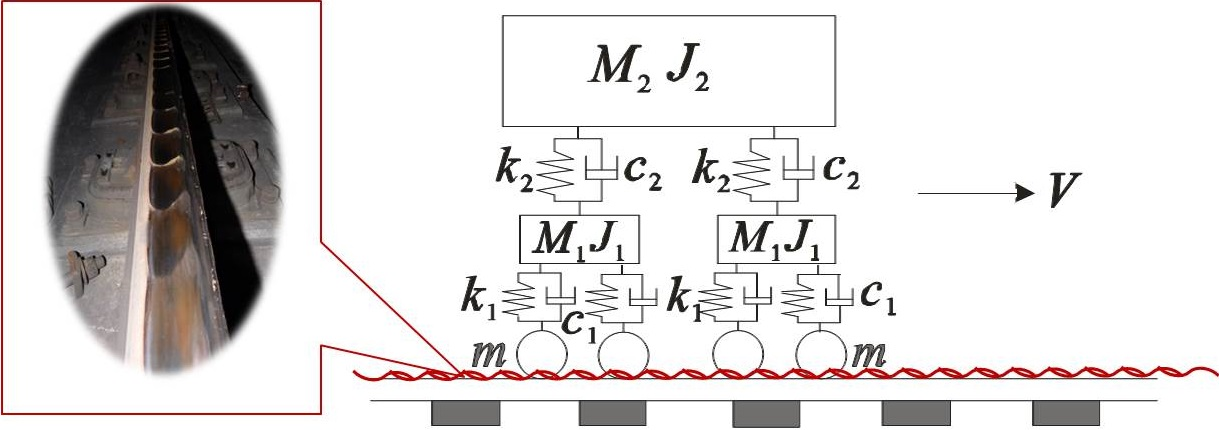#### Highlights

• Simulation on rolling noise and vibration generation in high frequency range needs considering "acoustic roughness"
• In low frequency range (<10 Hz), there is no obvious difference between US-PSD irregularity and perfect smooth rail
• Corrugated rail plays the dominant role on the vibration (about 250 Hz) which obviously related to its typical wavelength (63mm) at speed of 60km/h

## 1. Introduction

The deterioration of running surface, including out-of-round wheels and corrugated rails, as which typical wavelength less than 1000 mm, is also defined as ‘acoustic roughness’. In order to distinguish the contribution of running surface deterioration by wheels and rail respectively, out-of-round wheels moving on perfect smooth rail surface in different train speeds had already discussed in a companion paper .

Were different from out-of-round wheels that were commonly neglected in modeling, track irregularities play an important role on nearly all of the calculation models as the excitation element. When modeling the dynamic stability of train running, calculating the substructures subjected to the moving loads from railway trains [2-4], and so on, it would be sufficient using irregularities which was simplify generated from functions of power spectrum density (PSD) summarized from US or Germany railway main lines. However, it should be figured out that the wavelength of US-PSD was scoped from 1.524 m to 304.8 m, which highest effected frequency would be about 11Hz when the train’s speed at 60 km/h. Obviously, the common used track irregularities would not satisfy the requirement simulation on rolling noise and vibration generation, which interested frequency would be up to 80 Hz, 250 Hz [5, 6], and even 4000 Hz.

Rail corrugation is a periodic deterioration on running surface of rail (Fig. 2). Measurement data shows rail corrugation lead to 15 to 20 dB (A) pass-by noise increasing than normal condition. The enlarged vibration and boring squeal noise are complained by the resident living nearby, metro passengers and even the metro train drivers .

In this paper, the effect of rail roughness on the simulation modeling was discussed, considering a perfect round wheels moving on different irregularities rail surface, including perfect smooth rail, irregularities generated from a US PSD, measurement data from corrugated rails.

## 2. Irregularities input data

The SIMPACK-ABQUES simulation model was presented in the companion paper . As the only alternating quantity, the input wheel-rail interaction irregularities were introduced in this section.

Generally, it would be sufficient using irregularities which was simplify generated from US-PSD. In this paper, the Level 6 irregularity was generated in default SIMPACK package, using Eq. (1) and Table 1 parameters:

1
${S}_{v}\left(\mathrm{\Omega }\right)=\frac{k{A}_{v}{{\mathrm{\Omega }}_{c}}^{2}}{{\mathrm{\Omega }}^{2}\left({\mathrm{\Omega }}^{2}+{{\mathrm{\Omega }}_{c}}^{2}\right)}.$

And the final irregularities were shown in Fig. 1.

Table 1US-PSD track irregularity spectrum parameters

 ${\mathrm{\Omega }}_{c}$ rad/m ${\mathrm{\Omega }}_{s}$ rad/m ${A}_{a}$ cm2∙rad/m ${A}_{v}$ cm2∙rad/m Train speed (km/h) 0.8245 0.4380 $0.0$339 0.0339 60

Fig. 1Irregularities generated from US-PDF Level 6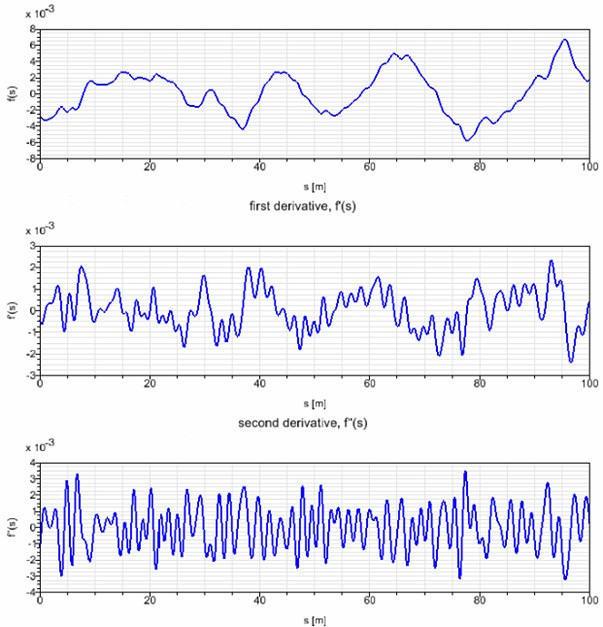### 2.2. Roughness from measured data for corrugated rail

For the corrugated rail, the roughness was measured using a corrugation analysis trolley (CAT) (Fig. 1). It is a hand operated device for measurement of longitudinal rail irregularities in the wavelength range from approximately 10 mm to 3,000 mm. Rail irregularities are determined by integrating the signal from a vertical accelerometer mounted on a hard steel ball which rolls on the rail. Another wheel with rubber coating is used to determine the longitudinal position of the trolley and to trigger the samples. The sampling distance was 1 mm. The data acquisition hardware was connected to a PC via a USB interface .

Fig. 2Corrugated rail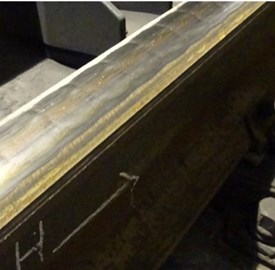Fig. 3Corrugation analysis trolley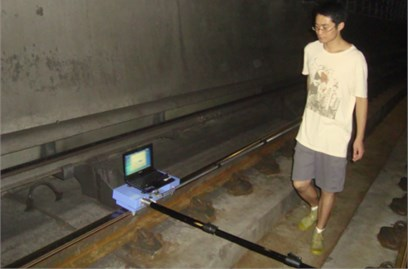The roughness data was captured from a measurement conducted in the section of DTVI2 track system in Beijing, in accordance with the model parameter. Raw data (Fig. 4) were provided as the input irregularities. The 1/3 octave wavelength spectrum was shown in Fig. 5, indicted that the typical wavelength of the measured corrugated rail was 63 mm.

Fig. 4Roughness of corrugated rail (Raw data)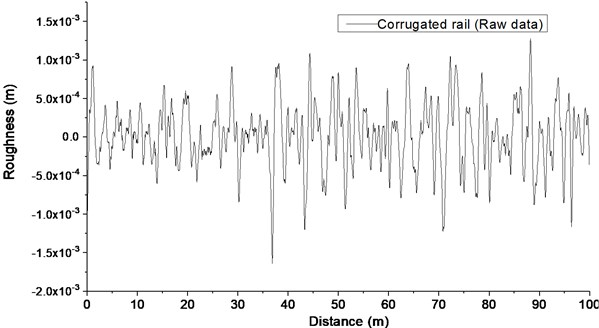Fig. 51/3 octave central wavelength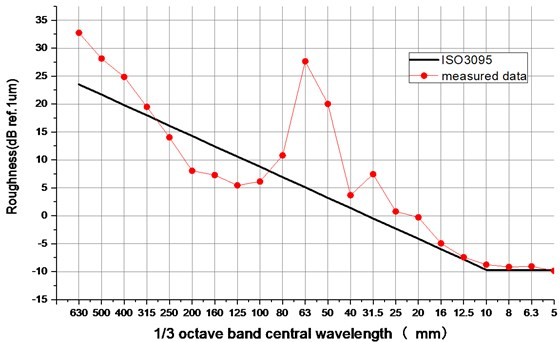## 3. Calculation result

The effect of rail roughness on the simulation modeling was discussed in this section, considering a train with perfect round wheels moved on different irregularities rail surface at the speed of 60 km/h, including perfect smooth rail, irregularities generated from a US PSD, measurement data from corrugated rails. As the rail vibration velocity always related to rolling noise, 1/3 octave band vibration velocity response were recorded and analyzed.

Fig. 6Rail velocity vibration for different irregularities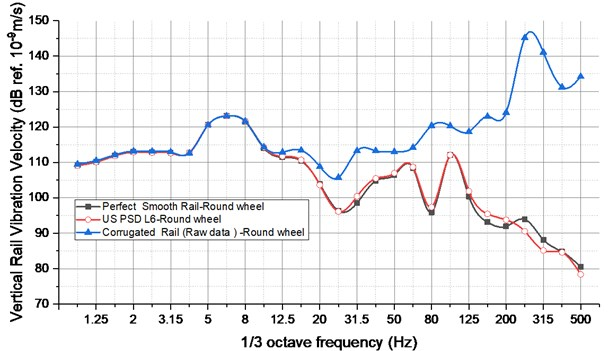From Fig. 6, it was clear that:

1) The curve of rail velocity vibration for using US PSD Lever 6 was very close to the irregularity of perfect smooth rail surface. It indicated the dynamic property of vehicle and track system plays the dominant role.

2) In low frequency range (less than 10 Hz), measurement irregularity data of corrugated rail was not made difference from perfect smooth rail;

3) In the frequency from 10 to 63 Hz, although the measurement irregularity data of corrugated rail raised the vibration velocity value, it still not alter the tendency of the curve that resulted by the dynamic property of vehicle and track system.

4) In high frequency that more than 63 Hz, the effect of corrugated rail would be not neglected any more, especially at the band of 250 Hz, corrugated rail plays the dominant role on the vibration which obviously related to the typical wavelength of 63 mm according to Eq. (2):

2

## 4. Conclusions

Based on the above presented simulation results, interesting findings could be summarized as following:

1) It was demonstrated that the irregularity generated from US-PSD would not satisfy the requirement simulation on rolling noise and vibration generation in high frequency range;

2) Corrugated rail plays the dominant role on the vibration which obviously related to its typical wavelength.

#### Acknowledgements

This work was under the support of Beijing Natural Science Foundation (No. 3184047), Beijing Academy of Science and Technology Funds (No.OTP-2018-002) and Beijing Public Finance innovation project in 2018(PXM2018-178304_000007).